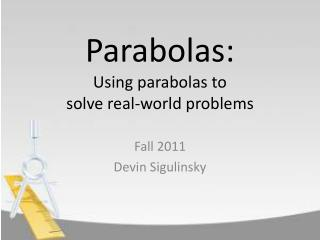DownloadDownload PresentationParabolas: Using parabolas to solve real-world problems

# Parabolas: Using parabolas to solve real-world problems

Télécharger la présentation## Parabolas: Using parabolas to solve real-world problems

- - - - - - - - - - - - - - - - - - - - - - - - - - - E N D - - - - - - - - - - - - - - - - - - - - - - - - - - -
##### Presentation Transcript

1. Parabolas:Using parabolas tosolve real-world problems Fall 2011 Devin Sigulinsky

2. Exercise Suppose a ball is thrown into the air. After seconds the ball travels a distance of feet. • What is the maximum height attained by the ball? • How long into the ball’s flight is this maximum height attained?

3. Back to Statement of Exercise Menu Algebraic Method Calculus Method Step C1 Step C2 Step C3 Step C4 Step C5 • Step A1 • Step A2 • Step A3 • Step A4 Compare and Contrast Methods So What?

4. Back to Statement of Exercise Step A1 Formula to find the x- coordinate of the vertex of a general parabola: Explanation of Step is a parabola. The coefficient on the term is negative, so the parabola opens downward. We need to find the vertex of the parabola. • -coordinate: Back to Menu

5. Back to Statement of Exercise Step A2 Find the -coordinate of the vertex of : Explanation of Step Identify the coefficients of corresponding to the coefficients in the formula from Step A1. Calculate the -coordinate. • ; • -coordinate is… Back to Menu

6. Back to Statement of Exercise Step A3 Find the time it takes for the ball to reach its maximum height: Explanation of Step The ball reaches a maximum height at seconds. At seconds, the ball reaches a maximum height of feet. Back to Menu

7. Back to Statement of Exercise Step A4 Conclusion: • The ball reaches a maximum height of feet after an elapsed time of seconds. Back to Menu

8. Back to Statement of Exercise Step C1 Overview of solution process: Reasoning behind overview of process. The maximum height reached by the ball occurs when the slope of the tangent line to the graph of is zero. The slope of the line tangent to the graph of is zero when the first derivative of equals zero. The zero of the first derivative corresponding to the slope of the tangent line is the time it takes for the ball to attain its maximum height. Evaluating at this zero yields the maximum height of the ball. • Find the first derivative of . • Find the zeros of the first derivative of . • Identify which zero of the first derivative of is a maximum for . This zero is the time at which attains its maximum value. • Calculate the height the ball reaches. Video Help Explaining Calculus Method in 3 Parts: Part 1Part 2Part 3 Back to Menu

9. Back to Statement of Exercise Step C2 Calculate the first derivative of : Explanation of Step Differentiate the function . Back to Menu

10. Back to Statement of Exercise Step C3 Calculate the zeros of the first derivative of : Explanation of Step To find the zeros of the first derivative of we set the first derivative equal to zero and solve for . is the only zero and so is the maximum, since is a parabola that opens downward. Back to Menu

11. Back to Statement of Exercise Step C4 Calculate the height the ball reaches: Explanation of Step The ball reaches its height after seconds, so evaluating at yields the maximum height of the ball. Back to Menu

12. Back to Statement of Exercise Step C5 Conclusion: • The ball reaches a maximum height of feet after seconds elapses. Back to Menu

13. Compare and Contrast Methods Algebraic Method Calculus Method 5 steps to complete the problem. Relatively more advanced math concepts. Method can be used with any function. • 4 steps to complete the problem. • Less advanced math concepts. • Method can only be used with parabolas. Back to Menu

14. So What? • Which method is “better”? Even though the algebraic method was easier, took less time, and there is a smaller chance of making arithmetic errors, the calculus method is far more versatile and probably a “better” method to use all around. Back to Menu